# K-means算法通俗原理及Python与R语言的分别实现

K均值聚类方法是一种划分聚类方法，它是将数据分成互不相交的K类。K均值法先指定聚类数，目标是使每个数据到数据点所属聚类中心的总距离变异平方和最小，规定聚类中心时则是以该类数据点的平均值作为聚类中心。

01K均值法原理与步骤

（1）、所有类别样本数等于总样本数，即每个类类是互不相同的（2）、每一类(假设是第i类)中数据点到聚类中心距离平方总和di为：

xi表示第i类各点平均值（聚类中心）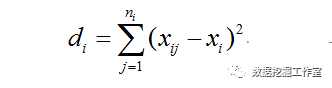（3）、K类数据点距离之和为：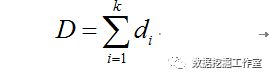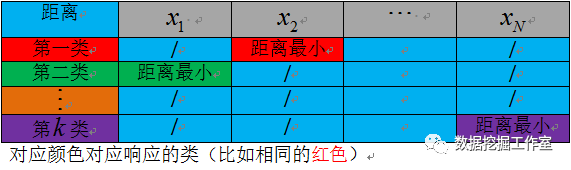K均值步骤：

1. 随机选取K个数据点作为（起始）聚类中心；
2. 按照距离最近原则分配数据点到对应类；
3. 计算每类的数据点平均值（新的聚类中心）；
4. 计算数据点到聚类中心总距离；
5. 如果与上一次相比总距离下降，聚类中心替换；
6. 直到总距离不再下降或者达到指定计算次数。

K-means算法缺点主要是：

1. 对异常值敏感；
2. 需要提前确定k值；
3. 结果不稳定；

02 K均值算法Python的实现

1. 首先用random模块产生随机聚类中心；
2. 用numpy包简化运算；
3. 写了一个函数实现一个中心对应一种聚类方案；
4. 不断迭代；
5. matplotlib包结果可视化。

```import numpy as np
import random as rd
import matplotlib.pyplot as plt
import math
#数据
dat = np.array([[14,22,15,20,30,18,32,13,23,20,21,22,23,24,35,18],
[15,28,18,30,35,20,30,15,25,23,24,25,26,27,30,16]])
print(dat)
#======聚类中心======#
n = len(dat)
N = len(dat)*n
k = 3
#-------随机产生-----#
center = rd.sample(range(n),k)
center = np.array([dat.T[i] for i in center])
print('初始聚类中心为：')
print(center)
print('-----------------------')

#计算聚类中心
def cent(x):
return(sum(x)/len(x))

#计算各点到聚类中心的距离之和
def dist(x):
#聚类中心
m0 = cent(x)
dis = sum(sum((x-m0)**2))
return(dis)

#距离
def f(center):
c0 = []
c1 = []
c2 = []
D = np.arange(k*n).reshape(k,n)
d0 = center-dat.T
d1 = center-dat.T
d2 = center-dat.T
d = np.array([d0,d1,d2])
for i in range(k):
D[i] = sum((d[i]**2).T)
for i in range(n):
ind = D.T[i].argmin()
if(ind == 0):
c0.append(i)#分配类别
else:
if(ind == 1):
c1.append(i)
else:
c2.append(i)
C0 = np.array([dat.T[i] for i in c0])
C1 = np.array([dat.T[i] for i in c1])
C2 = np.array([dat.T[i] for i in c2])
C = [C0,C1,C2]
print([c0,c1,c2])
s = 0
for i in C:
s+=dist(i)
return(s,C)

n_max = 50
#初始距离和
print('第1次计算！')
dd,C = f(center)
print('距离和为'+str(dd))
print('第2次计算！')
center = [cent(i) for i in C]
Dd,C = f(center)
print('距离和为'+str(Dd))
K = 3

while(K<n_max):
#两次差值很小并且计算了一定次数
if(math.sqrt(dd-Dd)<1 and K>20):
break;
print('第'+str(K)+'次计算！')
dd = Dd
print('距离和为'+str(dd))
#当前聚类中心
center = [cent(i) for i in C]
Dd,C = f(center)
K+=1

#---聚类结果可视化部分---#

j = 0
for i in C:
if(j == 0):
plt.plot(i.T,i.T,'ro')
if(j == 1):
plt.plot(i.T,i.T,'b+')
if(j == 2):
plt.plot(i.T,i.T,'g*')
j+=1

plt.show()```

（1）：聚类成功的例子：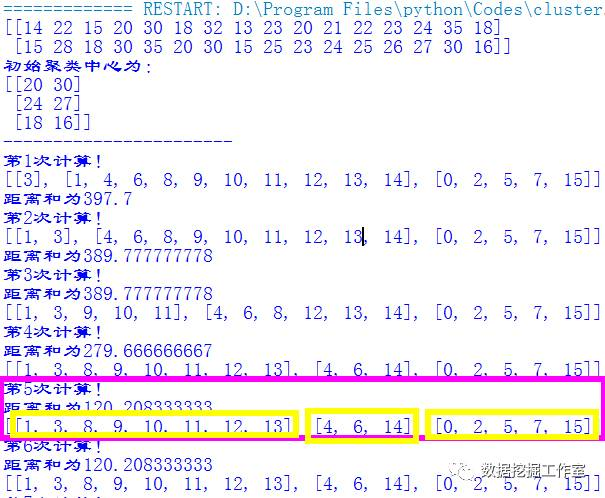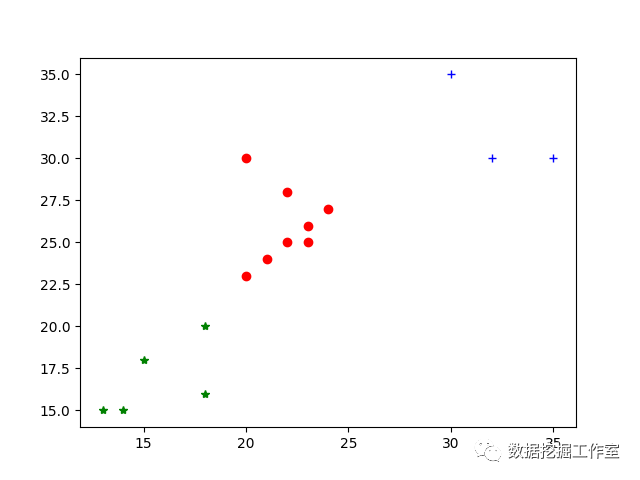（2）：聚类失败的例子：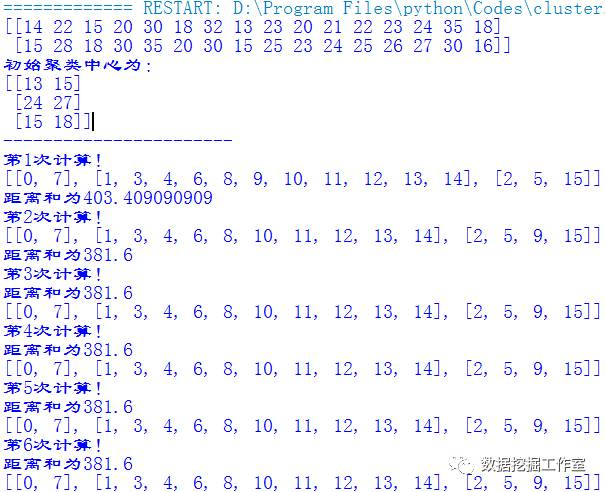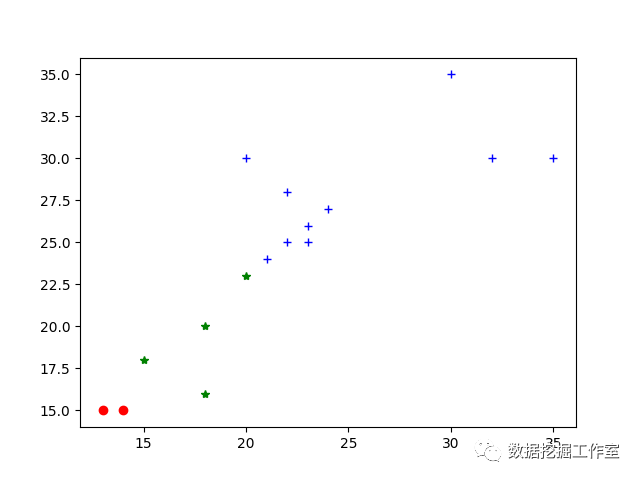03K均值算法的R语言实现

```rm(list = ls())
path <- 'C:\\Users\\26015\\Desktop\\clu.txt'
dat <- t(dat)
kc <- kmeans(dat,3)
summary(kc)
kc```

```K-means clustering with 3 clusters of sizes 8, 3, 5

Cluster means:
[,1]     [,2]
1 21.87500 26.00000
2 32.33333 31.66667
3 15.60000 16.80000```

Clustering vector:

V1  V2  V3  V4  V5  V6  V7  V8  V9

3   1   3  1   2   3   2   3  1

V10 V11 V12 V13 V14 V15 V16

1   1   1   1   1   2   3

• 本文由 发表
• 转载请务必保留本文链接：https://www.plob.org/article/10967.html